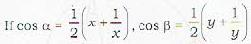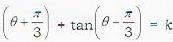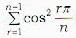Courses

# Test : Trigonometric Functions And Identities - 2

## 20 Questions MCQ Test Topic-wise Tests & Solved Examples for IIT JAM Mathematics | Test : Trigonometric Functions And Identities - 2

Description
This mock test of Test : Trigonometric Functions And Identities - 2 for Mathematics helps you for every Mathematics entrance exam. This contains 20 Multiple Choice Questions for Mathematics Test : Trigonometric Functions And Identities - 2 (mcq) to study with solutions a complete question bank. The solved questions answers in this Test : Trigonometric Functions And Identities - 2 quiz give you a good mix of easy questions and tough questions. Mathematics students definitely take this Test : Trigonometric Functions And Identities - 2 exercise for a better result in the exam. You can find other Test : Trigonometric Functions And Identities - 2 extra questions, long questions & short questions for Mathematics on EduRev as well by searching above.
QUESTION: 1

Solution:
QUESTION: 2

Solution:
QUESTION: 3

### If sinα + sinβ = α and cosα - cosβ = b then tanα - β/2 is equal to

Solution:
QUESTION: 4

If 0 < β < α < π/4 , cos(α + β) 3/5 and cos (α - β) = 4/5 then sin2α is equal to

Solution:
QUESTION: 5then cos(α-β) is equal to

Solution:
QUESTION: 6

If tanθ + tantan 3θ then K is equal to

Solution:
QUESTION: 7

If α secα - c tanα - d and b sec - d tanα = e then

Solution:
QUESTION: 8

If cos 20° - sin20° = p then cos 40° is equal to

Solution:
QUESTION: 9

If 3sinθ + 4cosθ = 5 then the value of 4sinθ 3cosθ is

Solution:
QUESTION: 10

If cos 2x + 2 cos x = 1 then sin2x (2 - cox2x) is equal to

Solution:
QUESTION: 11

If α, β, γ and δ be four angles of a cyclic quadrilateral then the value of cos α + cos β + cos y + cos δ is

Solution:
QUESTION: 12

if 4nα = π then cotα . cot2α . cot3α ... cot(2n -1)a is equal o

Solution:
QUESTION: 13

The value of cos12°.cos24°.cos36°.cos48°•cos72°.cos 84° is

Solution:
QUESTION: 14

The value of cosπ/11 + cos 3π/11+ cos 5π/11 + cos7π/ 11 + cos 9π/11 is

Solution:
QUESTION: 15is equal to

Solution:
QUESTION: 16

The number of distinct solutions of sin 5θ . cos 3θ = sin9θ • cos 7θ in [0,π/2] is

Solution:
QUESTION: 17

The number of solutions of sin2θ + 3cosθ = 3 in [-π,π] is

Solution:
QUESTION: 18

The number of distinct solutions of secθ + tanθ = √3, 0 ≤ θ, < 3π, is

Solution:
QUESTION: 19

The number of solutions of the equation 5secθ - 13 = 12tanθ in [0,2π] is

Solution:
QUESTION: 20

The equation p cos x - q sin x - r has solution for x only if

Solution: